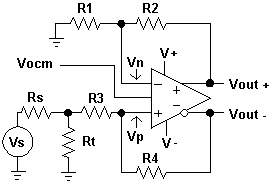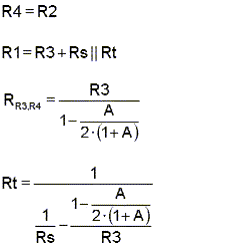Fully Differntial Amplifier Component Calculator

Problem:
What is the simplest way to calculate the values of components for an input-terminated single-ended to differential output amplifier.?

Solution:
FULLY DIFFERENTIAL AMPLIFIER COMPONENT CALCULATOR
 Inputs: Differential Gain W Rs value W R4 value W or R3 value W Resistors ExactE96 E192EquationsCalculated values Rt W R1 W R2 W R3 W R4 W
 Power and Input Voltages V+ V V- V Vs (DC) V Vocm V
 Resulting Gain V Resulting Voltages Vn V Vp V Vout+ V Vout- V
When a fully differential amplifier is employed, it is important to balance the two feedback paths with the same resistor ratio to prevent an offset from being present between the two outputs. When a single-ended input is to be terminated and connected to one of the inputs, this termination must be accounted for in the feedback ratio for that input. Doing this results in a gain change. In addition, the termination resistor value used should take into account the input impedance of the amplifier circuit. For these reasons, it is difficult to calculate optimum component values for a fully differential amplifier design whose input is single ended and terminated .

The JavaScript application above accepts your entry of source resistance (Rs), feedback resistor (R4) or gain resistor (R3) and desired differential gain (A). It then calculates the other resistors.

Selecting "E96" or "E192" radio buttons will cause the calculated resistor values to be selected from the E96 (96 values per decade) or E192 (192 values per decade) group respectively. Although E96 values are commonly know as 1% resistors while E192 values are known as 0.5% resistors, tighter tolerances are available. When the "Exact" radio button is selected the values are not selected from E96 or E192 groups.

RR3, R4 is the input imedance represented by R3 and a portion of R4 as determined by the action of the amplifier and its gain.

Limits:
1. The gain entry is the differential gain of the total circuit. The voltage divider consisting of Rs, Rt and the amplifier input resistance causes a gain of 0.5 at the input of R3. A gain of 2 from this point to the outputs will cause a total differential gain of 1. A gain entry of less than 0.5 is not allowed. A total gain less than 0.5 would cause the amplifier to be less than unity gain and therefore it may not be stable. The maximum gain entry is 1000.
2. The input impedance of the stage reflects the active response of the amplifier combined with the values of R3 and R4. The design is not valid if the stage input impedance is less than the source resistance.
3. Values entered for R3 and R4 must be positive numbers.
4. Resistor values, either entered or calculated must be equal to or between 1 W and 1 MW.